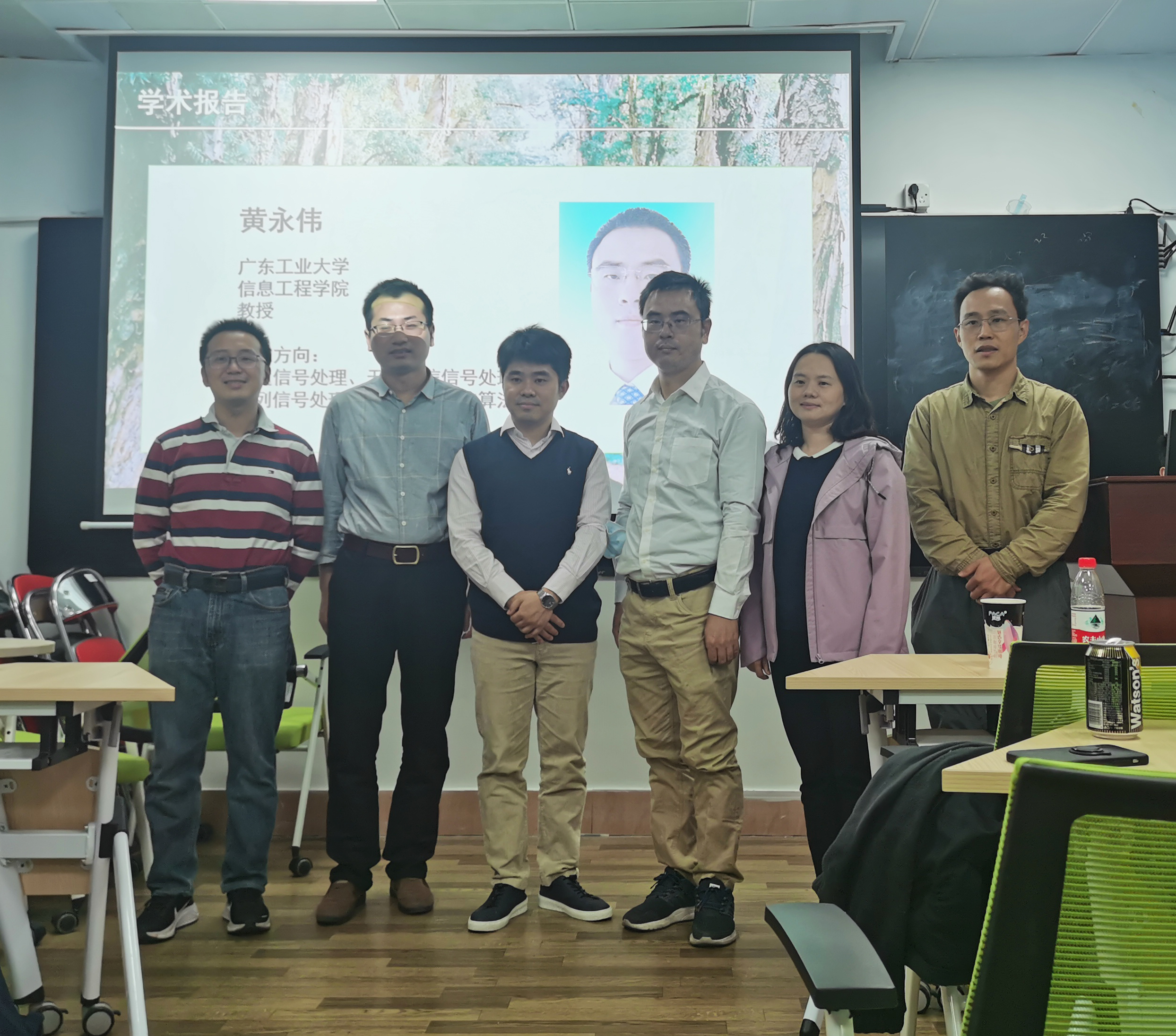Colloquiums and Conferences

# Quadratic Matrix Inequality Approach to Robust Adaptive Beamforming for General-Rank Signal Model

Time: 2021-11-16 10:18:00

Topic：Quadratic Matrix Inequality Approach to Robust Adaptive Beamforming for General-Rank Signal Model

Speaker：Yongwei Huang    Guangdong University of Technology

Time：2021-11-15  15:00--16:00

Location：Room401

Introduction：

The worst-case robust adaptive beamforming problem for general-rank signal model is considered. This is a nonconvex problem, and an approximate version of it (obtained by introducing a matrix decomposition on the presumed covariance matrix of the desired signal) has been well studied in the literature. Different from the existing literature, herein however the original beamforming problem is tackled. Resorting to the strong duality of linear conic programming, the robust adaptive beamforming problem for general-rank signal model is reformulated into an equivalent quadratic matrix inequality (QMI) problem. By employing a linear matrix inequality (LMI) relaxation technique, the QMI problem is turned into a convex semidefinite programming problem. Using the fact that there is often a positive gap between the QMI problem and its LMI relaxation, an approximation algorithm is proposed to solve the robust adaptive beamforming in the QMI form. Besides, several sufficient optimality conditions for the nonconvex QMI problem are developed. To validate our results, simulation examples are presented, which demonstrate the improved performance of the new robust beamformer in terms of the output signal-to-interference-plus-noise ratio.